# Curves and Surfaces

Curves and surfaces are objects like meshes, but differ in that they are expressed in mathematical functions, rather than a mere point-to-point basis.

Blender implements Bézier and Non Uniform Rational B-Splines (NURBS) curves and surfaces. Both these, though following different mathematical laws, are defined in terms of a set of "control vertices" defining a "control polygon". The way the curve and the surface are interpolated (Bézier) or attracted (NURBS) by these might seem similar, at a first glance, to Catmull-Clark subdivision surfaces.

Curves and surfaces have advantages and disadvantages compared to meshes. They are defined by less data, so they produce nice results with less memory usage at modelling time, whereas the demands increase at rendering time.

Some modelling techniques are only possible with curves, like extruding a profile along a path, but very fine control, as that available on a per-vertex basis on a mesh, is not possible.

Briefly, there are occasions in which Curves and surfaces are more advantageous than meshes, and occasions where meshes are more useful. Only experience, and the reading of these pages, can give an answer.

# Curves

This section will describe both Bézier and NURBS curves, and show a working example of the former.

## Béziers

Bézier curves are the most commonly used type for designing letters or logos. They are very important to understand since they are also widely used in animation, both as path for objects to move on and as IPO curves to change the properties of objects as a function of time.

A control point (vertex) of a Bézier curve consists of a point and two handles. The point, in the middle, is used to move the entire control point; selecting it will also select the other two handles, and allow you to move the complete vertex. Selecting one or two of the other handles will allow you to change the shape of the curve by dragging them.

Actually a Bézier curve is tangent to the line segment which goes from the point to the handle. The 'steepness' of the curve is controlled by the handle's length.

There are four types of handles (Figure 1):

• Free Handle (black). This can be used in any way you wish. Hotkey: HKEY (toggles between Free and Aligned);

• Aligned Handle (purple). These handles always lie in a straight line. Hotkey: HKEY (toggles between Free and Aligned);

• Vector Handle (green). Both parts of a handle always point to the previous handle or the next handle. Hotkey: VKEY;

• Auto Handle (yellow). This handle has a completely automatic length and direction. Hotkey: SHIFT+H.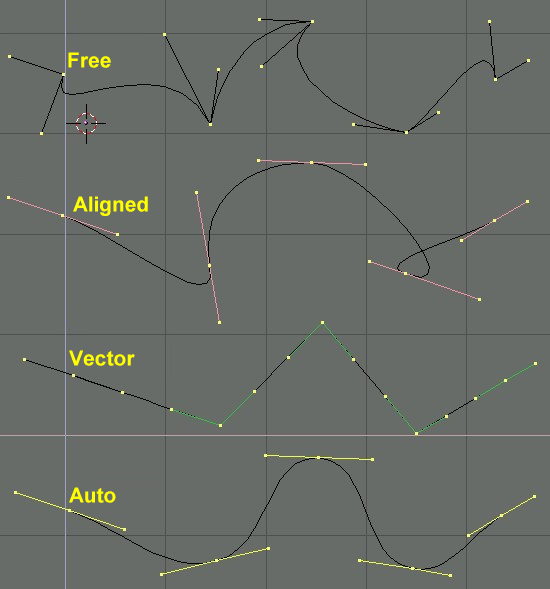Figure 1. Types of Handles for Bézier curves

Handles can be rotated by selecting the end of one of the vertices. Again, use the grabber with RMB-hold-move.

As soon as the handles are rotated, the type is modified automatically:

• Auto Handle becomes Aligned;

• Vector Handle becomes Free.

Although the Bézier curve is a continuous mathematical object it must nevertheless be represented in discerete form from a rendering point of view.

This is done by setting a resolution property, which defines the number of points which are computed between every pair of control points.

A separate resolution can be set for each Bézier curve (the number of points generated between two points in the curve - Figure 2).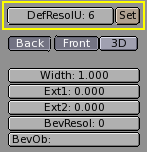Figure 2. Setting Bézier resolution.

## NURBS

NURBS curves are defined as rational polynomials, and are more general, strictly speaking, than conventional B-Splines and Bézier curves. They have a large set of variables, which allow you to create mathematically pure forms (Figure 3). However, working with them requires a little more intuition: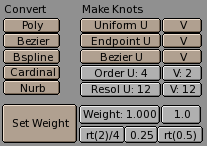Figure 3. Nurbs Control Buttons.

• Knots. Nurbs curves have a knot vector, a row of numbers that specifies the parametric definition of the curve. Two pre-sets are important for this. "Uniform" produces a uniform division for closed curves, but for open ones you will get "free" ends, difficult to locate precisely. "Endpoint" sets the knots in such a way that the first and last vertices are always part of the curve, hence much easier to place;

• Order. The order is the 'depth' of the curve calculation. Order '1' is a point, order '2' is linear, order '3' is quadratic, etc. Always use order '5' for Curve paths; this behaves fluidly under all circumstances, without irritating discontinuities in the movement. Mathematically speaking this is the order of both the Numerator and the Denominator of the rational polynomial defining the NURBS;

• Weight. Nurbs curves have a 'weight' per vertex - the extent to which a vertex participates in the "pulling" of the curve.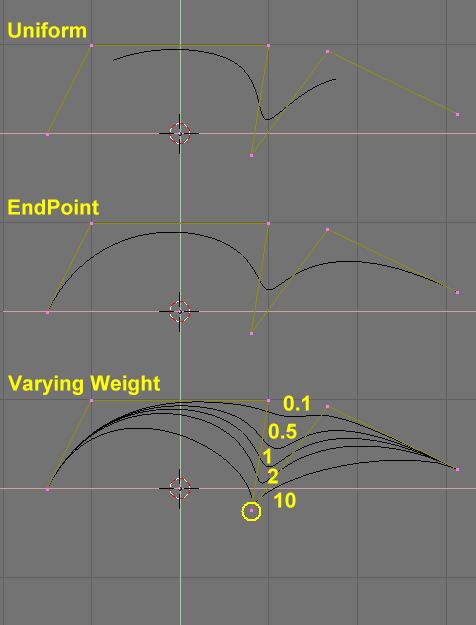Figure 4. Setting Nurbs Control polygon and weights.

Figure 4 shows the Knot vector settings as well as the effect of varying a single knot weight. Just as with Béziers, the resolution can be set on a per curve basis.

## Working example

Blender's curve tools provide a quick and easy way to build great looking extruded text and logos. We will use these tools to turn a rough sketch of a logo into a finished 3D object.

Figure 5 shows the design of the logo we will be building.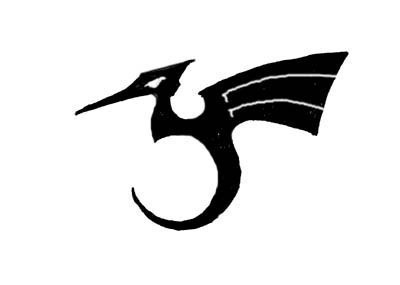Figure 5. The sketched logo

First, we will import our original sketch so that we can use it as a template. Blender supports TGA, PNG and JPG format images. To load the image, move the cursor over a 3D window and press SHIFT+F7 to get to the view settings for that window. Activate the BackGroundPic button and use the LOAD button to locate the image you want to use as a template (Figure 6).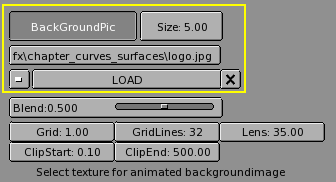Figure 6. 3D window settings.

Return to the 3D view by pressing SHIFT+F5 (Figure 7). You can hide the background image when you are finished using it by returning to the SHIFT+F7 window and deselecting the BackGroundPic button.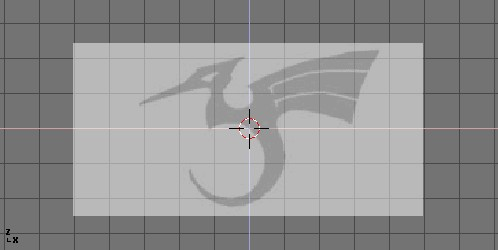Figure 7. Logo sketch loaded as background

Add a new curve by pressing SHIFT+A>>CURVE>>BEZIER CURVE. A curved segment will appear and Blender will enter EditMode. We will move and add points to make a closed shape that describes the logo you are trying to trace.

You can add points to the curve by selecting one of the two endpoints, then holding CTRL and clicking LMB. Note that the new point will be connected to the previously selected point. Once a point has been added, it can be moved by selecting the control vertex and pressing GKEY. You can change the angle of the curve by grabbing and moving the handles associated with each vertex (Figure 8).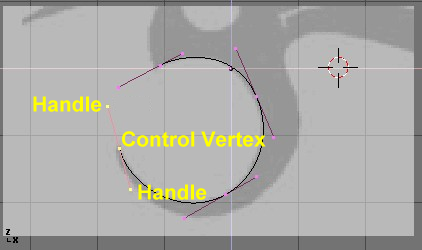Figure 8. Bézier handles

You can add a new point between two existing points by selecting the two points and pressing WKEY>>SUBDIVIDE (Figure 9).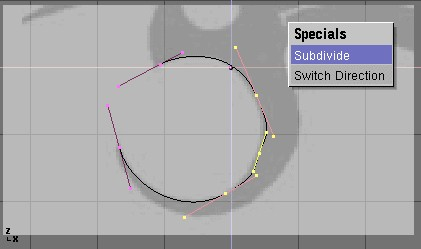Figure 9. Adding a Contrl Point.

Points can be removed by selecting them and pressing XKEY>>SELECTED. You cut a curve into two curves by selecting two adjacent control vertices and pressing XKEY>>SEGMENT.

To make sharp corners, you can select a control vertex and press VKEY. You will notice the colour of the handles change from purple to green (Figure 10). At this point, you can adjust the handles to adjust the way the curve enters and leaves the control vertex (Figure 11).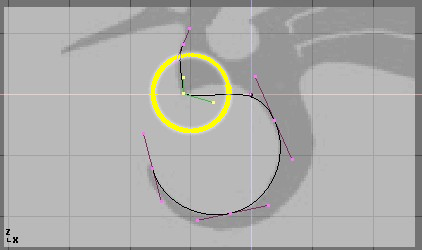Figure 10. Vector (green) handles.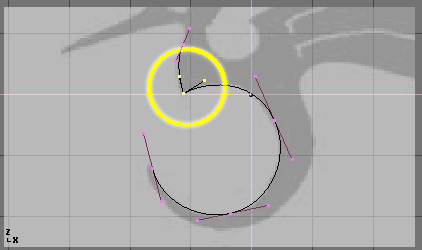Figure 11. Free (black) handles.

To close the curve and make it into a single continuous loop, select at least one of the control vertices on the curve and press CKEY. This will connect the last point in the curve with the first one (Figure 12). You may need to manipulate some more handles to get the shape you want.Figure 12. Figure Figure 8

Leaving editmode with TAB and entering shaded mode with ZKEY should reveal that the curve renders as a solid shape (Figure 13). We want to cut some holes into this shape to represent the eyes and wing details of the dragon. When working with curves, Blender automatically detects holes in the surface and handles them accordingly. Actually a closed curve is considered as the boundary of a surface. If a closed curve is completely included within another one, the former is subtracted by the latter, effectively defining a hole. Return to wireframe mode with ZKEY and enter editmode again with TAB.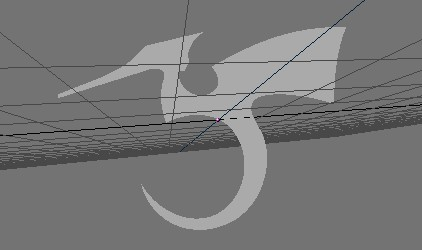Figure 13. Shaded logo.

While still in editmode, add a circle curve with SHIFT+A>>CURVE>>BEZIER CIRCLE (Figure 14). Scale the circle down to an appropriate size with SKEY and move it with GKEY.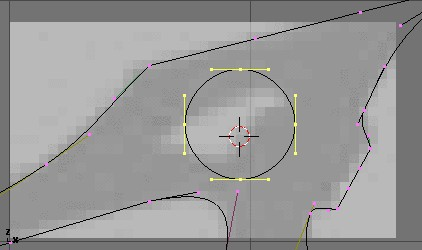Figure 14. Adding a circle.

Shape the circle using the techniques we have learned (Figure 15). Remember that you can add vertices to the circle with WKEY>>SUBDIVIDE.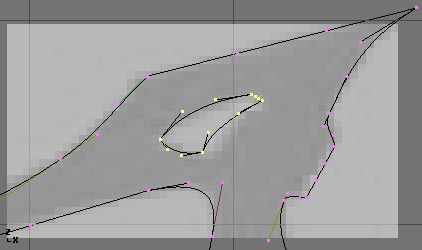Figure 15. Defining the eye.

Create a wing cutout by adding a Bézier circle, converting all of the points to sharp corners, and then adjusting as necessary. You can duplicate this outline to save time when creating the second wing cutout. To do this, make sure no points are selected, then move the cursor over one of the vertices in the first wing cutout and select all linked points with LKEY (Figure 16). Duplicate the selection with SHIFT+D and move the new points into position.Figure 16. Defining the wings.

If you want to add more geometry that is not connected to the main body (placing an orb in the dragon's curved tail for example), you can do this by using the SHIFT+A menu to add more curves as shown in Figure 17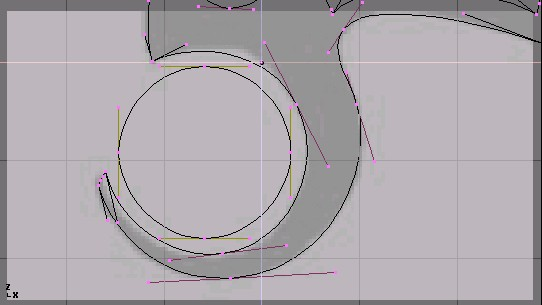Figure 17. Orb placement within the tail.

Now that we have the curve, we need to set its thickness and beveling options. With the curve selected, go to the EditButtons (F9). The "Ext1" parameter sets the thickness of the extrusion while "Ext2" sets the size of the bevel. BevResol sets how sharp or curved the bevel will be.

Figure 18 shows the settings used to extrude this curve.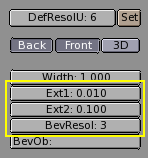Figure 18. Bevel settings

 From Curves to MeshesIf want to perform more complex modeling operations, you can convert the curve to a mesh with ALT+C>>MESH. Note that this is a one-way operation: you cannot convert a mesh back into a curve.

When your logo model is complete, you can add materials and lights and make a nice rendering (Figure 19).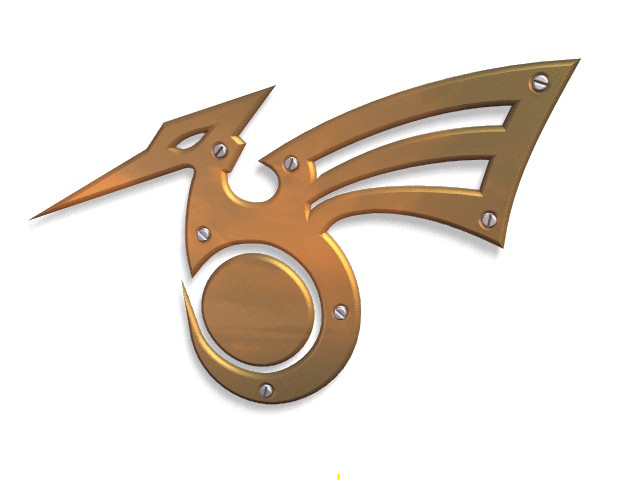Figure 19. Final rendering.